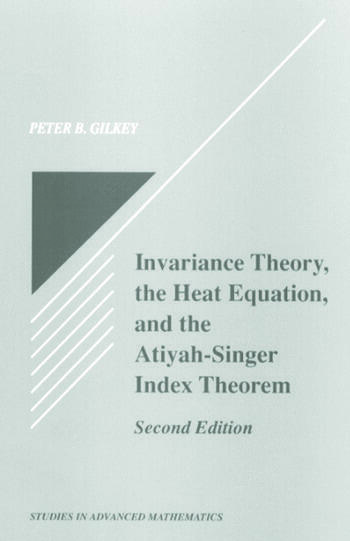Invariance Theory: The Heat Equation and the Atiyah-Singer Index Theorem

1st Edition

Peter B. Gilkey

CRC Press
Published December 22, 1994
Reference - 536 Pages
ISBN 9780849378744 - CAT# 7874

For Instructors Request Inspection Copy

USD\$300.00

FREE Standard Shipping!

Preview

Summary

This book treats the Atiyah-Singer index theorem using the heat equation, which gives a local formula for the index of any elliptic complex. Heat equation methods are also used to discuss Lefschetz fixed point formulas, the Gauss-Bonnet theorem for a manifold with smooth boundary, and the geometrical theorem for a manifold with smooth boundary. The author uses invariance theory to identify the integrand of the index theorem for classical elliptic complexes with the invariants of the heat equation.

Instructors

We provide complimentary e-inspection copies of primary textbooks to instructors considering our books for course adoption.